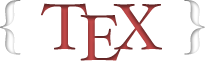## Distribution of scores on my answers

17

(click on this box to dismiss)

Shows how often a user's answers get a specific score. Related to http://odata.stackexchange.com/stackoverflow/q/1930Q&A for users of TeX, LaTeX, ConTeXt, and related typesetting systems

``````-- Distribution of scores on my answers
-- Shows how often a user's answers get a specific score. Related to http://odata.stackexchange.com/stackoverflow/q/1930

SELECT @totalAnswers = COUNT(*) FROM posts WHERE posttypeid = 2 AND owneruserid = ##UserId##

CASE WHEN Frequency < 1 THEN '<1%' ELSE Cast(Cast(ROUND(Frequency, 0) AS INT) AS VARCHAR) + '%' END AS Frequency
FROM (
SELECT score, COUNT(*) AS Occurences, (COUNT(*) / @totalAnswers) * 100 AS Frequency
FROM posts
WHERE posttypeid = 2                 -- answers
AND owneruserid = ##UserId##       -- by you
GROUP BY scoreHold tight while we fetch your results